# Crypto

Practical Math

## Elijah Caine M. Voigt

• DevOps Engineer at Nordstrom
• Recent BS in CS from Oregon State
• Blog https://elijahcaine.me1. Diffie Hellman Key Exchange
2. Elliptic Curve Crypto
3. Hash Functions
4. Memory Hard Functions
5. Secure multi-party computations
6. Garbled Circuits

## Diffie Hellman Key Exchange

A protocol for exchanging public keys over an insecure channel.

## Diffie Hellman Key Exchange

1. Alice and Bob publicly agree on a mod (p) and a base (g).
2. Alice and Bob both choose secret integers (a and b).
3. Alice sends Bob ga (mod p) (we call it A). Bob sends Alice gb (mod p) (we call it B).
4. Alice computes Ba (mod p). Bob computes Ab (mod p). These are equivalent (mod p). This is Alice and Bob's shared secret.

Ab = (ga)b = (gb)a = Ba (mod p)

Computing 'a' given 'A' is very difficult when 'a' is large

## Elliptic Curve Crytography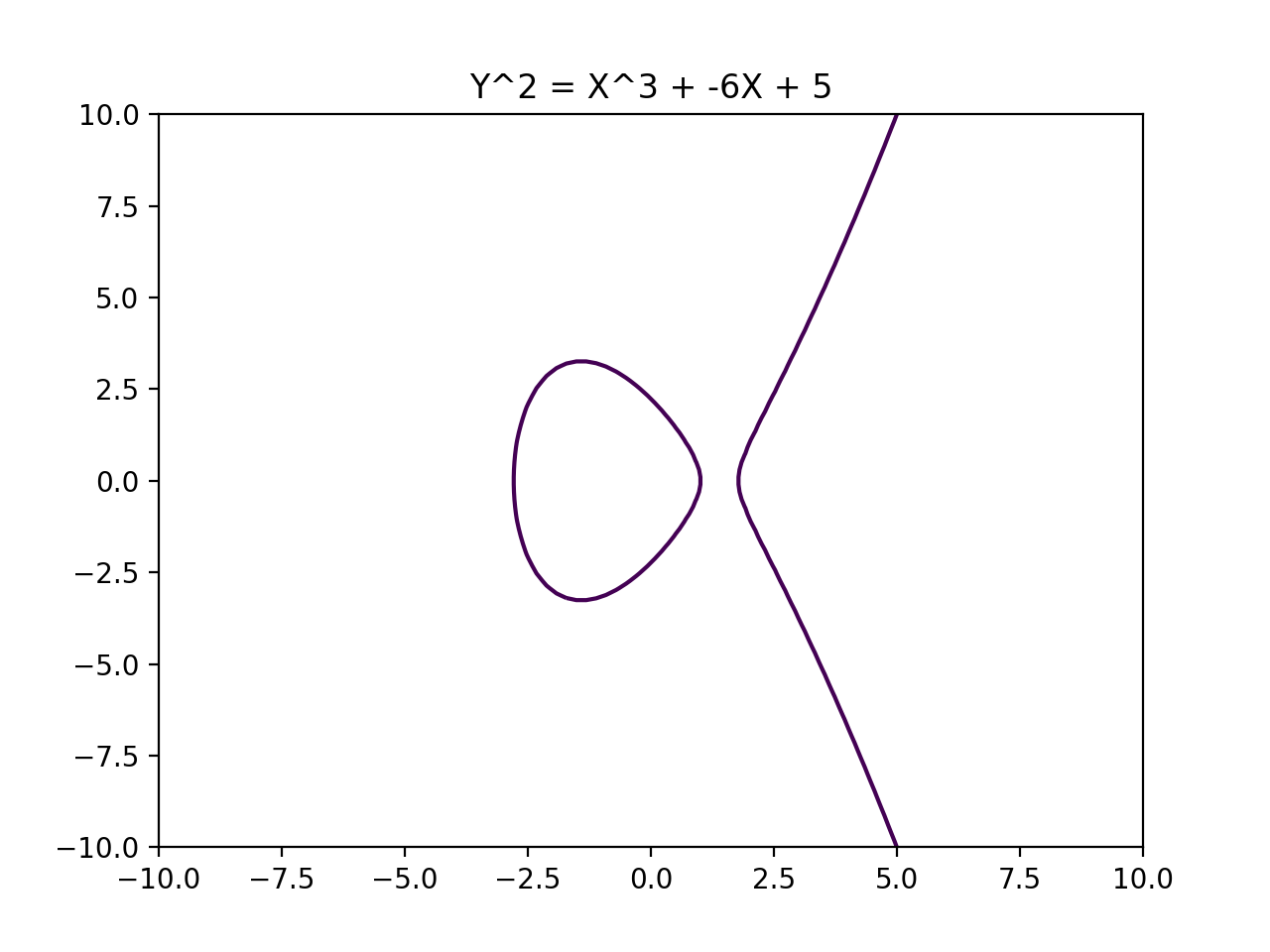### ECC Defined

Public Key Cryptography which uses special properties of Elliptic Curves to create shared secrets.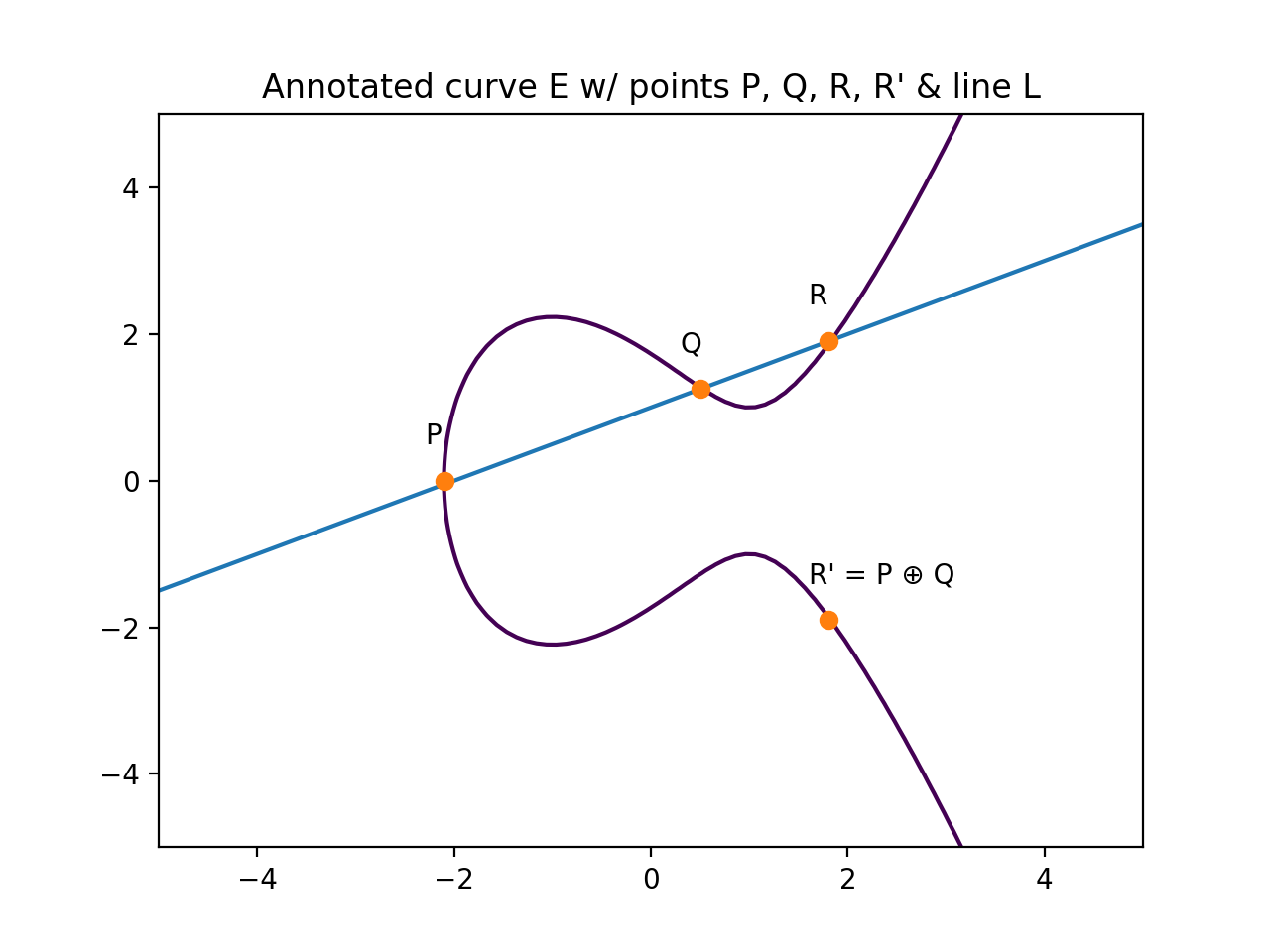1. Take two points P and Q on the Elliptic Curve E.
2. Draw a line L which passes through these two points.
3. L should ultimately pass through three points: P, Q, and R.
4. Multiply the Y coordinate of R by -1, this is R'.
5. P ⊕ Q = R'.

### "Multiplication"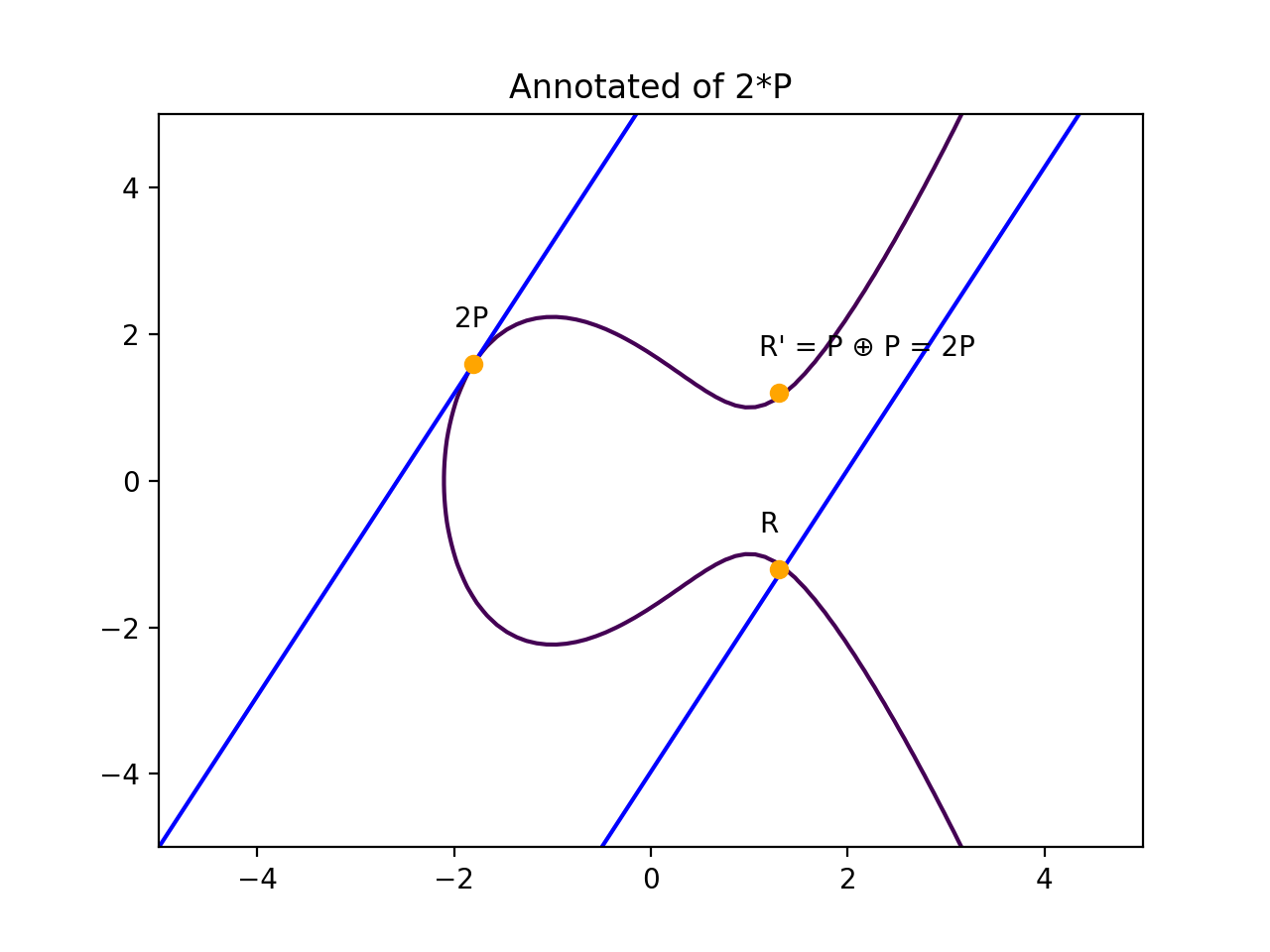### "Multiplication"

1. Take a point P ∈ E(Fp) and an integer n ≥ 1.
2. Set Q = P and R = O.
3. Loop while n > 0.
1. If n ≡ 1 (mod 2), set R = R + Q
2. Set Q = 2Q and n = floor(n/2).

### DHKE in ECC

1. Alice and Bob agree on E(Fp): an Elliptic Curve over a finite field and P A public point P on E(Fp).
2. Alice chooses a secret integer a and Bob choose secret integers b .
3. Alice computes Qa = a P and Bob computes Qb = b P. These are the "Public Keys"
4. Alice sends Bob her public key, Bob send Alice his public key.
5. Alice computes a Qb, Bob computes b Qa.
6. The shared secret is a Qb = a (b P) = b (a P) = b Qa.

### Why we care about ECC

Greater security with less data.

[...] breaking a 228-bit RSA key requires less energy than boiling a teaspoon of water.

[...] breaking a 228-bit elliptic curve key requires enough energy to boil all the water on Earth.

### In the wild

Check your TLS Certs for `_ECDHE_`

```Technical Details
Connection Encrypted (TLS_ECDHE_RSA_WITH_AES_128_GCM_SHA256, 128 bit keys, TLS 1.2)
```

## Hash Functions

A function that takes arbitrary size input and returns unique constant size output.

## Hash Functions

```H("Mary had a little lamb"): 8774a25d2f

H("Pop"): 21129ffe98

H("Mary had a little lamb"): 8774a25d2f
```

### Reversing a Hash Function

```H("password"): 6b3a55e026

H("123456"): e150a1ec81

H("hunter2"): 46a9d5bde7

...

H("qwerty"): 10231785cb
```

### Attempts to secure Hash Functions

```H(H(H(...H("SALTpassword")...))): c07e32d739

H(H(H(...H("SALT123456")...))): e0209a9bf0

H(H(H(...H("SALThunter2")...))): 3f02b38713

...

H(H(H(...H("SALTqwerty")...))): b2bb8650b7
```

### In the wild

Cryptographic Hash Functions:

Algorithm Secure?
MD5 No
SHA-1 No
SHA-256 No-ish
SHA-512 Yes-ish
SHA-3 Yes

Note

Fun Fact: The SHA family of hash functions were developed by the NSA!

## Memory Hard Functions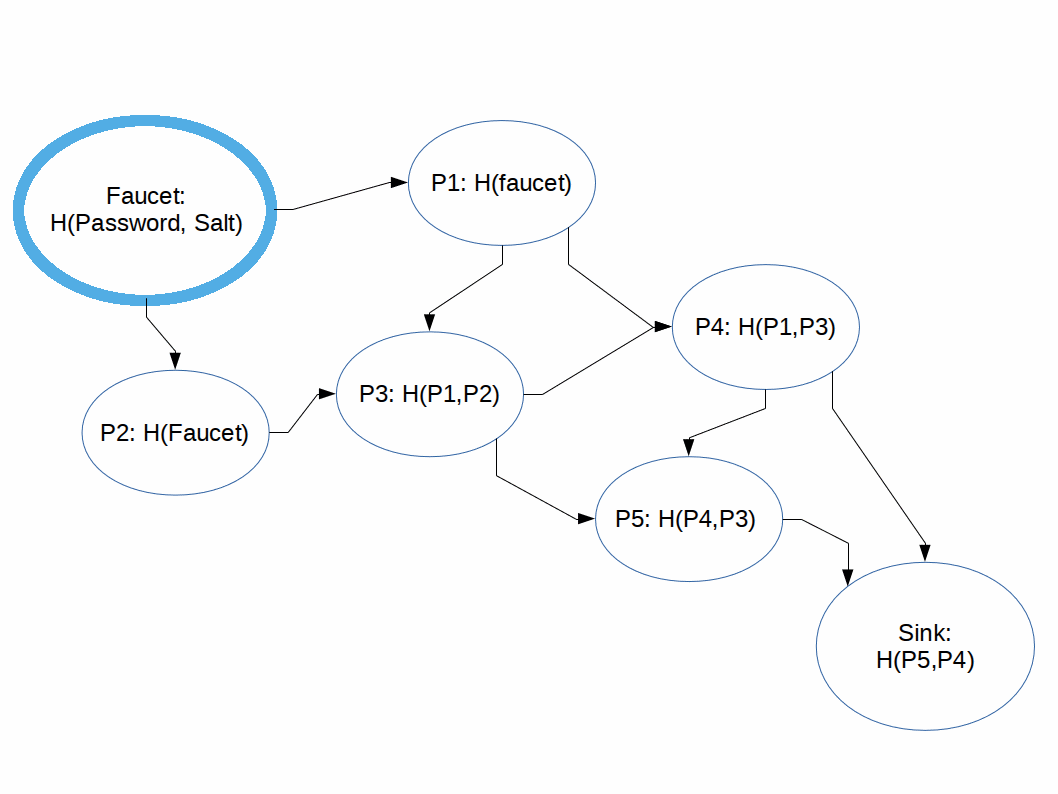### MHFs Defined

Hashing functions which are difficult to compute in memory-constrained systems.

### Directed Acyclic Graphs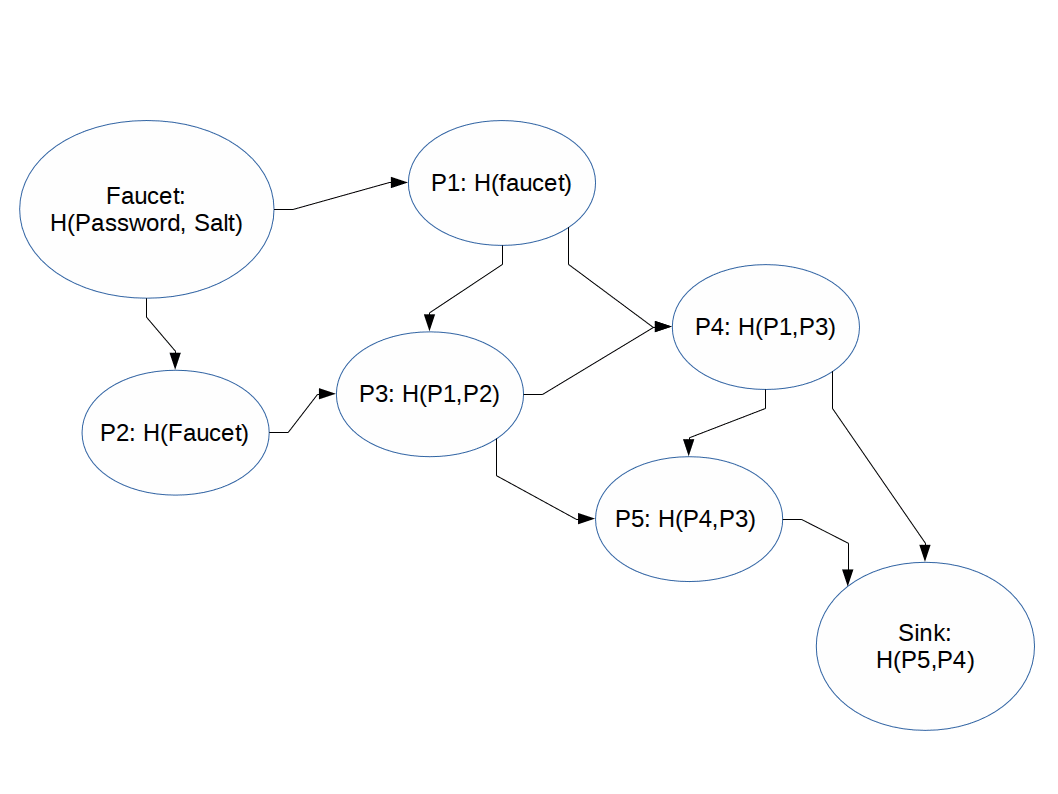### Directed Acyclic Graphs### Why we care about MHFs

Before:

```ASIC Hash Function Compute Time: 4 cycles (concurrent)
EC2 Hash Function Compute Time: 20 cycles
```

Memory Hard:

```ASIC Memory Hard Hash Compute Time: 20+ cycles (sequential)
EC2 Memory Hard Hash Compute Time: 20 cycles
```

Scrypt

## Secure multi-party computation

Evaluation of a program without knowledge of it's inputs or contents.

## Secure multi-party computation

The Millionare Problem

```function F = garbled(function f)
input A = obfuscated(input a)
input B = obfuscated(input b)
F(A,B) = f(a,b)
```

## Garbled Circuits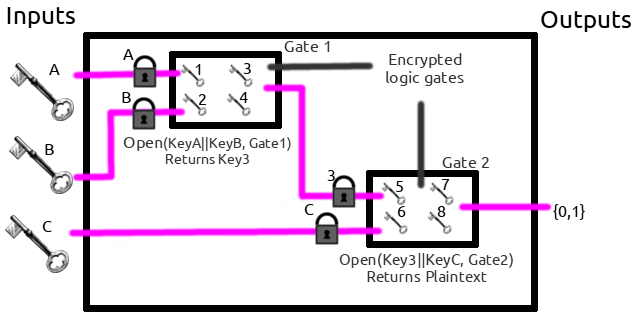### Garbled Circuits defined

An implementation of secure multi-party calculations.

### How to Garble

OR 0 1
0 0 1
1 1 1
1. Four possible inputs, Four possible outputs.
2. Inputs are encrypted ('0' -> 4355a46b19, etc)
3. Two keys used to decrypt one gate outputs.
4. Seeng inputs (encryption keys) doesn't spoil the values (`1` or `0`).
5. One can run the circuit without knowing the inputs.

Note

Now let's walk through an example where Alice and Bob will garble and evaluate an "OR" gate.

### Alice's circuit & input

1. Alice generates 4 keys `Kx0`, `Kx1`, `Ky0`, and `Ky1`.
2. Alice creates four variables corresponding with the four outputs of the OR table:

Plaintext

OR 0 1
0 0 1
1 1 1

Garbled

OR `Kx0` `Kx1`
Ky0 `B00` `B01`
Ky1 `B10` `B11`

### Alice's circuit & input

1. Each box is encrypted with the two keys:
OR Kx0 Kx1
Ky0 `E( Kx0||Ky0, B00 )` `E( Kx0||Ky1, B01 )`
Ky1 `E( Kx1||Ky0, B10 )` `E( Kx1||Ky1, B11 )`

Which looks like:

OR `f4982f5cfd4` `56198d52cb1`
`abfff1c5ed8` `d9b2aefb1fe` `d56f56bb9bb`
`abc7b0fb361` `917df3320d7` `4f2a86deb5e`
1. Alice sends the ciphertexts (`E( Kx0||ky0, B00)`, etc) and her input (`KxA`) to Bob.

### Bob's input

1. Bob takes Alice's input(s), `KxA`.
2. Bob uses oblivious transfer to compute is input `KyB`.
3. Bob can run the circuit. `garbled_OR( KxA , KyB )` outputs 0 or 1.

Note

Bob has enough information to get one of the four possible outputs of the circuit, but doesn't know if Alice's input is a 1 or a 0 at each circuit.

Importantly, while Bob can share the output of the circuit, he should not share his input. That would make using OT (step 6) obtuse.

Contact sharing.

Note

Good question.

### Why it all mattersNote

This matters for a few reasons:

THESIS THESIS THESIS???